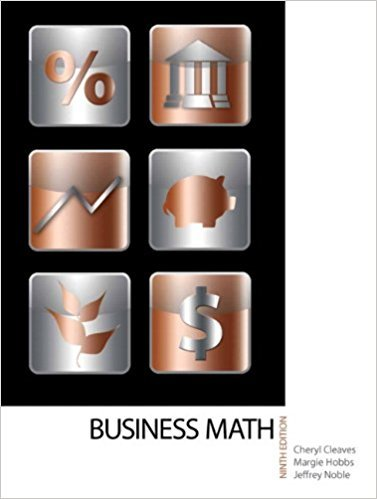×
×

# Solutions for Chapter 5-1: EQUATIONS## Full solutions for Business Math, | 9th Edition

ISBN: 9780135108178Solutions for Chapter 5-1: EQUATIONS

Solutions for Chapter 5-1
4 5 0 408 Reviews
21
1
##### ISBN: 9780135108178

Since 36 problems in chapter 5-1: EQUATIONS have been answered, more than 18078 students have viewed full step-by-step solutions from this chapter. This expansive textbook survival guide covers the following chapters and their solutions. Chapter 5-1: EQUATIONS includes 36 full step-by-step solutions. This textbook survival guide was created for the textbook: Business Math, , edition: 9. Business Math, was written by and is associated to the ISBN: 9780135108178.

Key Math Terms and definitions covered in this textbook
• Adjacency matrix of a graph.

Square matrix with aij = 1 when there is an edge from node i to node j; otherwise aij = O. A = AT when edges go both ways (undirected). Adjacency matrix of a graph. Square matrix with aij = 1 when there is an edge from node i to node j; otherwise aij = O. A = AT when edges go both ways (undirected).

• Affine transformation

Tv = Av + Vo = linear transformation plus shift.

• Basis for V.

Independent vectors VI, ... , v d whose linear combinations give each vector in V as v = CIVI + ... + CdVd. V has many bases, each basis gives unique c's. A vector space has many bases!

• Circulant matrix C.

Constant diagonals wrap around as in cyclic shift S. Every circulant is Col + CIS + ... + Cn_lSn - l . Cx = convolution c * x. Eigenvectors in F.

• Cross product u xv in R3:

Vector perpendicular to u and v, length Ilullllvlll sin el = area of parallelogram, u x v = "determinant" of [i j k; UI U2 U3; VI V2 V3].

• Diagonalizable matrix A.

Must have n independent eigenvectors (in the columns of S; automatic with n different eigenvalues). Then S-I AS = A = eigenvalue matrix.

• Free columns of A.

Columns without pivots; these are combinations of earlier columns.

• Krylov subspace Kj(A, b).

The subspace spanned by b, Ab, ... , Aj-Ib. Numerical methods approximate A -I b by x j with residual b - Ax j in this subspace. A good basis for K j requires only multiplication by A at each step.

• Left inverse A+.

If A has full column rank n, then A+ = (AT A)-I AT has A+ A = In.

• Linear transformation T.

Each vector V in the input space transforms to T (v) in the output space, and linearity requires T(cv + dw) = c T(v) + d T(w). Examples: Matrix multiplication A v, differentiation and integration in function space.

• Linearly dependent VI, ... , Vn.

A combination other than all Ci = 0 gives L Ci Vi = O.

• Nullspace matrix N.

The columns of N are the n - r special solutions to As = O.

• Nullspace N (A)

= All solutions to Ax = O. Dimension n - r = (# columns) - rank.

• Partial pivoting.

In each column, choose the largest available pivot to control roundoff; all multipliers have leij I < 1. See condition number.

• Positive definite matrix A.

Symmetric matrix with positive eigenvalues and positive pivots. Definition: x T Ax > 0 unless x = O. Then A = LDLT with diag(D» O.

• Simplex method for linear programming.

The minimum cost vector x * is found by moving from comer to lower cost comer along the edges of the feasible set (where the constraints Ax = b and x > 0 are satisfied). Minimum cost at a comer!

• Special solutions to As = O.

One free variable is Si = 1, other free variables = o.

• Spectral Theorem A = QAQT.

Real symmetric A has real A'S and orthonormal q's.

• Subspace S of V.

Any vector space inside V, including V and Z = {zero vector only}.

• Vector v in Rn.

Sequence of n real numbers v = (VI, ... , Vn) = point in Rn.

×